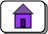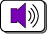created: 07/04/96; 2nd edition: 05/26/01; additions: 09/25/01; audio: 09/20/03; revised 05/20/15, 02/11/21CHAPTER 8 — Binary Addition and Two's Complement

Digital computers use bit patterns to represent many types of data. Various operations can be performed on data. Computers perform operations on bit patterns. With a good representation scheme, bit patterns represent data and bit pattern manipulations represent operations on data.

The Binary Addition Algorithm inputs two bit patterns representing integers to create a third pattern which represents the sum of the integers.

Chapter Topics:

• Sign-magnitude Representation
• Two's complement Representation
• Overflow detection in unsigned binary
• Overflow detection in two's complement binary

Most processor chips implement the Binary Addition Algorithm in silicon as part of their arithmetic logic unit. In a course in digital electronics you will study the hardware details of its implementation. This chapter discusses the fundamentals of the algorithm.

QUESTION 1:

Compute the following. Give the answer in binary notation.

0 + 0 = ?
0 + 1 = ?
1 + 0 = ?
1 + 1 = ?